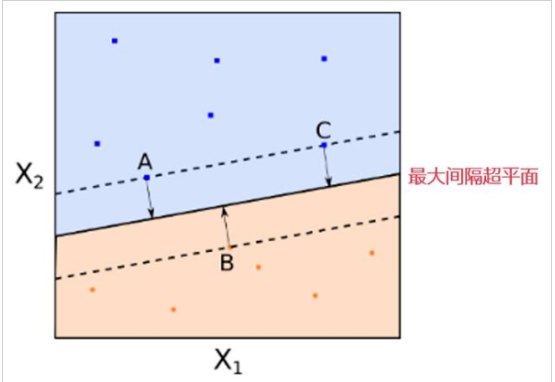1 数学基础

1.1 什么是支持向量？

1.2 什么是超平面？

1.3 超平面数学方程

1.4 点到平面的距离

1.5 函数间隔和几何间隔

2 SVM内容

2.1 SVM的数学表达式

2.2 对偶问题

2.3 拉格朗日函数

2.4 拉格朗日乘子法

3 扩展服务

3.1 扩展服务

# 什么是支持向量？

## 1、列向量的表示法

（1）小写黑斜体字母表示法：$\bold{a}$。如果表示行向量，则需要加$\bold{a}^T$。

（2）箭头表示法：$\vec{a}$。

（3）集合-元素表示法，如下所示：

$$X = \begin{bmatrix} x_1 \\ x_2 \\ \vdots \\ x_n \end{bmatrix}$$

$$X = \begin{bmatrix} x_1 \ x_2 \ ... \ x_n \end{bmatrix} ^T$$

## 2、支持向量